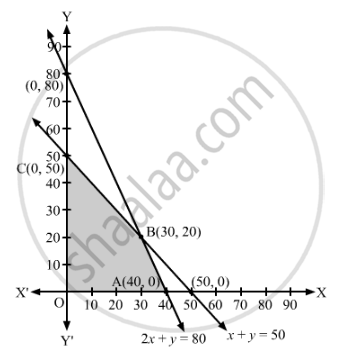# A Cooperative Society of Farmers Has 50 Hectares of Land to Grow Two Crops X and Y. the Profits from Crops X and Y per Hectare Are Estimated as ₹10,500 and ₹9,000 Respectively. - Mathematics

Sum

A cooperative society of farmers has 50 hectares of land to grow two crops X and Y. The profits from crops X and Y per hectare are estimated as ₹10,500 and ₹9,000 respectively. To control weeds, a liquid herbicide has to be used for crops X and Y at the rate of 20 litres and 10 litres per hectare, respectively. Further not more than 800 litres of herbicide should be used in order to protect fish and wildlife using a pond which collects drainage from this land. How much land should be allocated to each crop so as to maximise the total profit of the society?

#### Solution

Let the land allocated for crop X be x hectares and crop Y be y hectares.

Maximum area of the land available for two crops is 50 hectares.

∴ x + y ≤ 50

The liquid herbicide to be used for crops X and Y are at the rate of 20 litres and 10 litres per hectare, respectively. Maximum amount of herbicide to be used is 800 litres.

∴ 20x + 10 y ≤ 800

⇒ 2x + y ≤ 80

The profits from crops X and Y per hectare are ₹10,500 and ₹9,000, respectively.

Thus, total profit = ₹(10,500x + 9,000y)

Thus, the given linear programming problem is

Maximise Z = 10500x + 9000y

subject to the constraints

x + y ≤ 50

2x + y ≤ 80

≥ 0, y ≥ 0

The feasible region determined by the given constraints can be diagrammatically represented as,The coordinates of the corner points of the feasible region are O(0, 0), A(40, 0), B(30, 20) and C(0, 50).

The value of the objective function at these points are given in the following table.

 Corner Points Z = 10500x + 9000y (0, 0) 10500 × 0 + 9000 × 0 = 0 (40, 0) 10500 × 40 + 9000 × 0 = 420000 (30, 20) 10500 × 30 + 9000 × 20 = 495000 (0, 50) 10500 × 0 + 9000 × 50 = 450000

So, the maximim value of Z is 495000 at x = 30 and y = 20.

Therefore, 30 hectares of land should be allocated for crop X and 20 hectares of land should be allocated for crop Y.

The maximum profit of the society is ₹4,95,000.

Concept: Graphical Method of Solving Linear Programming Problems
Is there an error in this question or solution?

#### APPEARS IN

RD Sharma Class 12 Maths
Chapter 30 Linear programming
Exercise 30.4 | Q 42 | Page 55